# 和屌丝一起学SWIFT-基础运算符

[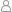孤狼 |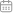2015-12-02 10:29:09 |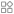Swift |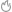2261°C |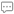0条评论 ]本教程仅用于初学SWIFT同学使用，内容由本人（孤狼）学习过程中笔记编写，本教程使用SWIFT版本为2.0。本教程内容可以自由转载，但必须同时附带本声明，或注明出处。PAEA.CN版权所有。

大家好,又见面了,欢迎回到和屌丝一起学SWIFT系列教程,上节我们说了SWIFT基础,这节课我们一起看看SWIFT里的运算符.基本上都是一些常见的运算符,有过编程基础的人这节课都可以直接跳过的哦.[赋值运算符]

``var a = 123;print(a);``

[算术运算符]

``print(1+1); //等于2print(1-1); //等于0print(2*2); //等于4print(2/2); //等于1``

TIP:当+号的两边不是数字类型,而是字符串则做拼接处理.

``print("你好"+"坏"); //输出 "你好坏"``

TIP:当运算符的两边类型不一致时,无法运算

``print(1+"哈哈");  //报错``

[求余运算符]

``print(10%3);    //等于1print(10%2.6);  //等于2.2``

[自增与自减]

Swift 也提供了对变量本身加1或减1的自增( ++ )和自减( -- )的缩略算符

``var a = 1;a++;print(a);   //等于2``

``var b=1;var c=2;print(b++ * c); //等于2[先计算,后自增]print(++b * c); //等于6[先自增,后计算]``

[一元负号]

``var a = -1; //如果你这里运行错误,请留意文章最后的小贴士.print(a-1); //等于-2``

[一元正号]

[符合赋值符]

Swift提供把其他运算符和赋值运算( = )组合的组合赋值运算符,比如 +=,-=,*=,/=

``var a = 12,b = 12,c = 12,d = 12;a += 1;     //a=a+1;print(a);   //等于13b -= 2;     //b=b-2print(b);   //等于10c *= 2;     //c=c*2print(c);   //等于24d /= 2;     //d=d/2print(d);   //等于6``

[比较运算符]

[三目运算符]

``条件?真结果:假结果;``
``var a = (2>1) ? "大于" : "小于";print(a);   //结果:大于``

[区间运算符]

``for i in (2...9){   print(i);   //结果23456789}``

``for i in (2..<9){   print(i);   //结果2345678}``

[逻辑运算符]

``if(2>1||1>2){   print("真");}else{   print("假");}``

``if(2>1&&1>2){   print("真");}else{   print("假");}``

``if(!(2>1)){   print("真");}else{   print("假");}``

``if(2>1||1>2||1>3||1>4){   print("真");}else{   print("假");}``

``if(2<1&&1<2&&1<3&&1<4){   print("真");}else{   print("假");}``

小贴士:在SWIFT中,定义或传值的时候,记得留个空格,SWIFT不会向其他语言一样对空格不敏感,比如,var a=-1; 就会报错,需要写成 var a = -1;所以,要注意留空格.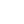赞赏一个鸡腿